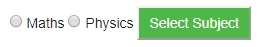# Passing Radio Button Data to CGI Program in Python

Radio Buttons are used when only one option is required to be selected.

## Example

Here is example HTML code for a form with two radio buttons −

<form action = "/cgi-bin/radiobutton.py" method = "post" target = "_blank">
<input type = "radio" name = "subject" value = "maths" /> Maths
<input type = "radio" name = "subject" value = "physics" /> Physics
<input type = "submit" value = "Select Subject" />
</form>

The result of this code is the following form −Below is radiobutton.py script to handle input given by web browser for radio button −

#!/usr/bin/python
# Import modules for CGI handling
import cgi, cgitb
# Create instance of FieldStorage
form = cgi.FieldStorage()
# Get data from fields
if form.getvalue('subject'):
subject = form.getvalue('subject')
else:
subject = "Not set"
print "Content-type:text/html\r\n\r\n"
print "<html>"
print "</html>"